Part III Phi and BeyondLet us return to viewing this essential odd-even split in the alef-bet as a proportion. We can draw them as two adjoining segments on a line, two adjacent rectangles, or even as we have represented them in the 3-d bar graph below. The important thing is the proportion, not the shape that is being split apart; it is the relationship between the two shapes.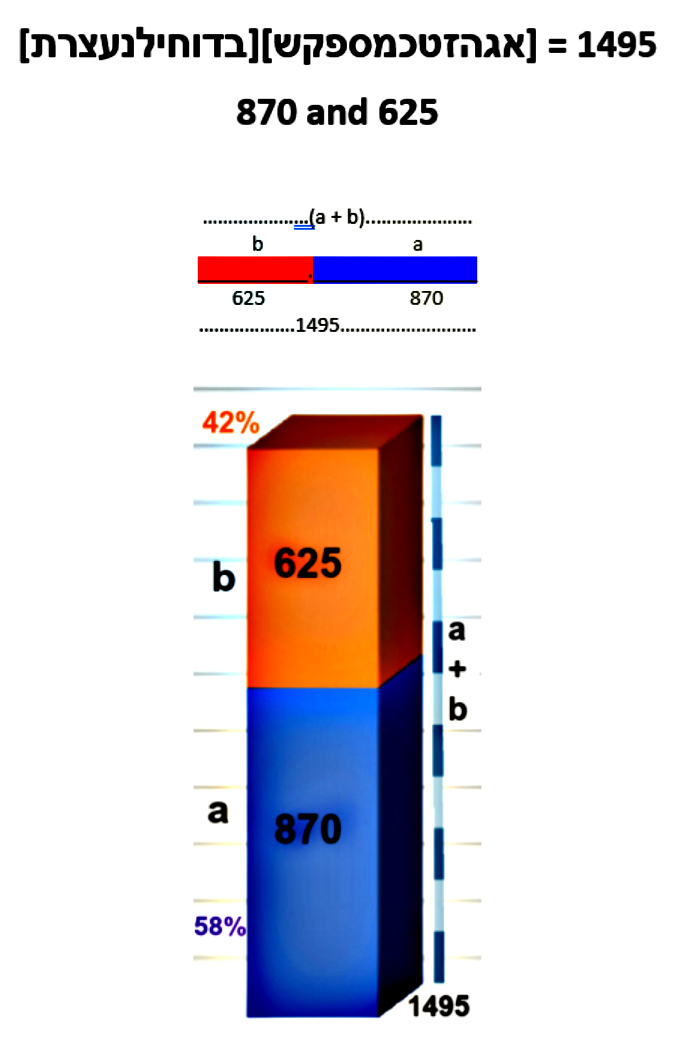We know that the odd set is 72% of the even set and that the odd set is 42% of the whole set of letters. Mathematically, we write this as b : a = .7180 and b : c = .4180

b : a = .7180

b : (a+b) = .4180

What is this reminiscent of? The Golden Mean, Phi().

b : a = .6180

a : (a + b) = .6180

The relationship between the segments a to b and between the segments b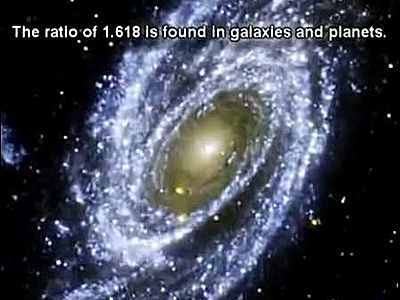to (a + b) is highly reminiscent to the harmonic golden mean, the Phi () proportion. Phi() is a mathematical constant, an irrational number that naturally and automatically guides the most efficient and beautiful spiraling growth in the universe.  This includes flower petals, seashells, tree branches, the shape of our bodies, our DNA, the galaxies in the universe and several different aspects of black holes.  It is not a stretch to say that without Phi() chaos would truly reign in our universe, as will be explained later. For now, just picture your DNA instead of following neat exact double helix spirals, as random knots, or the petals on a flower as a jumbled mess.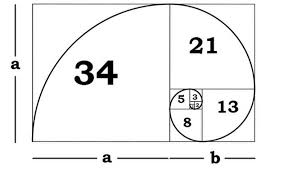Quite simply, the Phi () proportion is such that the small segment (b) is to the larger one (a) as the larger one is to the overall length (a + b). This proportion is called Phi () and is equivalent to .61803399….. The spiraling growth happens by continually dividing the larger segment by .618033…, or alternatively multiplying it by 1.618033…

If we were to apply the Phi () proportion to the 1495 total value of the alef-bet, the subordinate values would be split into 924 + 571 = 1495, where a = 924 and b = 571.

1495 x .6180 = 924

924  x  .6180 = 571

1495

Comparing the Phi () proportioned alphabet to the odd-even split we can see that it is an exact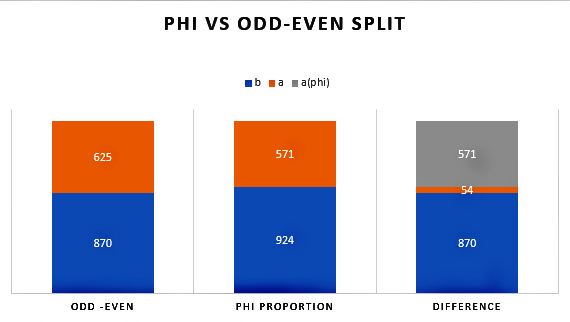shift of 54 units from Phi () to the Odd/Even split.

625 – 54 = 571

870 + 54 = 924

Why so exact? If we review the proportions, we recall that:

625/1495 was .4180

625/870 was   .7183

They are off from Phi (), .6180 by a pretty round number:

.6180 – .4180 = .2000

.7183 – .6180 = .1003

A Connection to Power and the Torah’s First Verse

Oddly enough, when we add the digits in the odd/even split we get:

6 + 2 + 5 + 8 + 7 + 0 = 28

We also get 28 when we add the digits in the Phi() split:

5 + 7 + 1 + 9 + 2 + 4 = 28

And again, when we add the total value of the alef-bet plus the shift of 54 units:

1 + 4 + 9 + 5 + 5 + 4 = 28Summing up the digits may seem like child’s play compared to the rest of the mathematics we are discussing, yet it is a typical part of Number Theory, which is one of the main branches of mathematics.  As will be obvious soon, the number 28 here relates to the 28 letters in the Torah’s first verse. The fact that all three equations, dealing with the four different components, sum to 28 is not slight of hand. There is no reason mathematically for them to work out that way; it must either be a tremendous coincidence or purposeful design.  The beauty of Number Theory is that it deals in hard facts and can reveal and/or disprove wonderful symmetries in the framework of our universe.

The same question—a tremendous coincidence or purposeful design—can similarly be applied to the sum total of the standard gematria value for all 304,805 letters in the Torah: 21009826. The sum of its digits (2+1+0+0+9+8+2+6) = 28, the same result (28) that we saw in the equations above.  Also, the product of those same digits gives us (2*1*0*0*9*8*2*6) = 1728.  Then applying another deeply revealing form of gematria cipher by dividing the above product by the above sum, we get:

4√(P(21009826)/ ∑(21009826)) = 4√(1728/28) = 2.802828

This is not so dissimilar from the square root of the exact number of words in the Torah: 282.8002828

You see, pure facts, just like 2 + 2 = 4, revealing beautiful symmetry. Though this does not prove the existence of G-d, it does go a long way in proving purposeful design, which can be taken as divine intent. Now some scholars in both Number Theory and ancient Kabbalistic gematria studies would go one step further in all these equations and reduce 28 to 2 + 8 = 10, which further reduces to 1 + 0 = 1.  More on that later.  In case you were wondering, the number 28 is the gematria value for the Hebrew word Koach(כח), meaning “power.”

Here are a couple more pure facts about the total gematria of the Torah. If you recall, we found the square root the total gematria sofit value (33640626) of the 304,805 letters in the Torah is 5800.054, which was astonishing considered that exact collective number of words, letters and verses in the Torah is exactly 58. Nevertheless, the gematria sofit value and the standard gematria (21009826) value are also tied to those of 58 quantitative (or structural) elements of the Torah through its square root, 625, which also represents the proportion 5/8 = .625:

21009826/33640626 ≈ .625 = 5/8.

Moreover, while the sum of the 22 spelled out Hebrew letters is 4248, it becomes 10048 when the final letters are considered in the spelling, in other words exactly (10048-4248) = 5800 more.  Oh, and by the way, (3+3+6+4+0+6+2+6) + (2+1+0+0+9+8+2+6) = 58.  Did any of this have to work out this way? No. Could it have happened accidentally, by random chance? No. Well yes, there is exactly one way it could work out by random chance and that is if there exists an infinite set of varying universes and we just happen to live in the One that everything concerning our Torah, and as you will soon see, everything concerning our mathematical and physical constants, our mathematical constructs and the dimensions of our solar system also happen to work out with perfect symmetric harmony.  Either way, it makes us pretty special.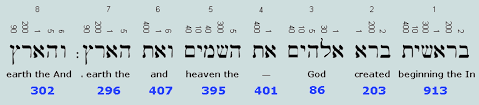Nonetheless, back to that first 28 letter verse of the Torah, the first 5 letters (בראשי) of that verse and thus of the Torah have a collective ordinal value, letter placement within the alphabet value, of (2+20+1+21+10) = 54.

Please bear with me as I reveal yet another secret code embedded into the Torah. I know it is out of place, but I just noticed a heretofore unforeseen pattern and cannot contain my excitement. If we plot out the ordinal values of the first 11 letters of the Torah. Notice that it is 11 letters again, and please note that there are exactly 11 unique numbers in that same first verse. A hint in unraveling the Torah encoding is that whenever there is abundant redundancy, someone is trying to communicate a message to us and that it is important enough to ensure we do not miss it. Plotting out those 11 letters, we get:

בראשית־ברא־אל

2,20,1,21,10,22,2,20,1,1,12 and their sum is 112.

First, we combine the letters so you can see what I was seeing:

22012110222201112

Then we break them down to natural viable triplets:

BRE  Sh  Y T      BRE   EL

If because all these letters just happened to work out to 0’s, 1’s and 2’s we can then convert this message from base 3 to base 10, giving us:

73  7 3 8 73 14

Permuting the letters slightly we get:

BRE  YSh  T   BRE   EL

Which translates to “G-d created Something 22”, implying not only G-d Created the 22 letters, but moreover, the deep kabbalistic concept of “Something from Nothing” that we will get into later.

BRE  Y – Sh – T   BRE   EL

We already know that the entire first verse totals 2701, which is the sum of all the integers from 1 – 73 and that 73 is the gematria of Cochma(חכמה), meaning “Wisdom,” and the second highest of the 11 dimensions. That total 2701 also equals 73 x 37.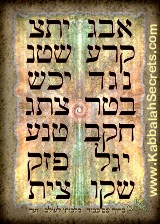If we broke the word sequence into its natural triplet sequencing (BRE, ShYT, BRE, EL) we would get (7+3+6+0+2+7+3) = 28, as is also (BRE YSH T = 7+3+7+3+8).  Meanwhile, there are 9 digits in (737387314) and 9 divided by the small gematria of the 42-Letter Name is 9/.123049… = 73.141593… combining 73 and Pi to an astonishing degree of accuracy. This 314 is also found in the Torah’s first word, (שית), again as the small gematria value (314). While it is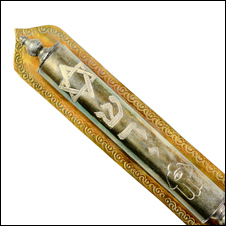obviously indicative of Pi (3.14…) it is also representative of the Name of G-d, Shadai (שדי) that we put on our doorposts, gateways and entrances.  That is all we are going to say about this today, the gateway to Wisdom and Knowledge. Moreover, reinforcing the “G-d Created Something 22”:

2+2+0+1+2+1+1+0+2+2+2+2+0+1+1+1+2 = 22 (letters)

Also, YeSh (יש) in base 6 is 54 and base 8 is 42, while in base 13 it is 28, all very relevant as we will soon see, as is Y- SH-T (י־ש־ת) or 378, the value of the famous phrase “Echad Ushmo Echad (אחד־ושמו־אחד)”  or “His Name is One.”

Yet if we kept things simple, and just used the 11 ordinal values as their individual base 10 equivalents, we would get:

2 + 6 + 1 + 7 + 3 + 8 + 2 + 6 + 1 + 1 + 5 = 42

We would have also seen 26, representing the Tetragrammaton (יהוה);
173, representing the small gematria value of the 42-Letter Name and the 173 Keys to Heaven the 1900-year-old Zohar tells us were given to Moses; and 26 yet again. And in all fairness to gematria buffs, 115 is the value of hazak (חזק), the Hebrew word for strength.

We digressed again, and yet it is interesting how this phenomenon of 28 connects to the resultant ratio: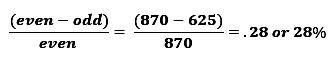Phi and 54-Unit Shift

The shift between the odd-even split of the alphabet and the Phi() one is exactly 54 units, so reminiscent of the 54 portions in the Torah.  Meanwhile, the 54-unit shift represents 5.844% of the larger Phi() split (924), which is so close to the 5845 verses in those 54 portions.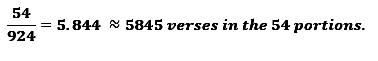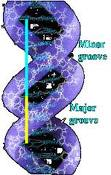That the Torah’s 54 portions and 5845 verses would be connected to Phi() is not surprising, considering that the first chapter of the Book of Numbers (Bamidbar) is the dividing point in the Torah in terms of the number of letters, the numbers of words, and also the number of verses into the exact Phi() proportion, just like the major and minor twists of our DNA: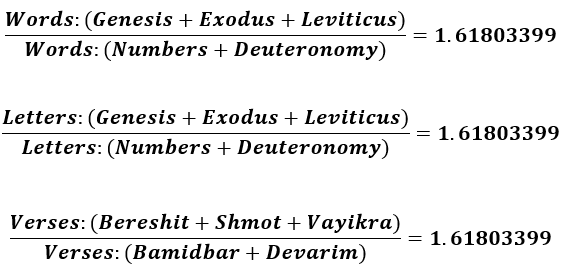Moreover, oddly enough, that first chapter of Bamidbar, where that telling split in the Torah occurs, has 54 verses, a wonderful synergistic connection to the 54-unit Phi() shift. The first verse of the Torah is also split into two sections that incorporate the number 54. We already illustrated a connection between 73 x 37, now we see that the last two words of that verse have a joint gematria value of 703, which is 19 x 37, while the first 5 words have a gematria of 1998 or 54 x 37.

What all these numbers have in common is that they all reduce to One (1).

73 →7 + 3 = 10  →   1 + 0 = 1 (One)

37→3 + 7 = 10  →   1 + 0 = 1 (One)

2701→ 2 + 7 + 0 + 1 = 10 →   1 + 0 = 1 (One)

19 → 1 + 9 = 10  →   1 + 0 = 1 (One)

(54 x 37) → 5 + 4 + 3 + 7 = 19 → 1 + 9 = 10 → 1 + 0 = 1 (One)

(19 x 54) → 1 + 9 + 5 + 4 = 19 → 1 + 9 = 10 → 1 + 0 = 1 (One)

And

541 (Israel) 5 + 4+ 1 = 10  →  1 + 0 = 1 (One)

424 (Moshiach Ben David) 4 + 2 + 4 = 10  →  1 + 0 = 1 (One)

The number 37 is in actuality 111/3 or the numerical value of Alef/3 so theGenesis 1:1 equation above translates to: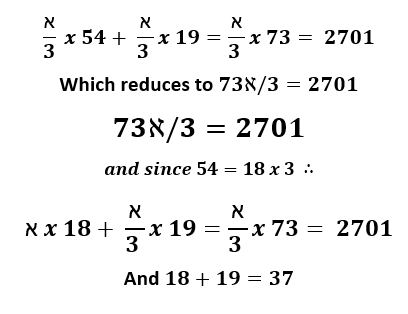Much has been written about the unique geometry of this first verse, though the only one we will mention today is that 37 and 73 are both consecutive 12-gonal numbers, meaning they form a filled in Magen David, Star of David.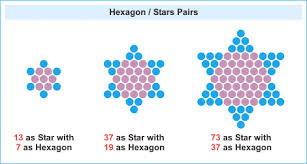The first two Star numbers are 1 and 13–harking back to Echad (One)–of the star number sequence is 1, 13, 37, 73…253…541, 661…. Most fittingly, the number 541, the numerical value of Israel, is the 10th Star number, while the 11th Star number, 661, is the gematria of Ester, the heroine of Purim, in which by the way, there are exactly 54 Hamans, her adversary in the Scroll of Ester.  Interestingly, the 7th Star number, 253, is the sum of all the integers from 1 – 22, as in the 22 letters.  Moreover, we have already explained that 73 is the value of the sefira Chochma, meaning Wisdom, not we can reveal that 37 is the ordinal value of Chochma, or inner Wisdom, which geometrically makes sense since because the central hexagon within the Star of 73 is 37.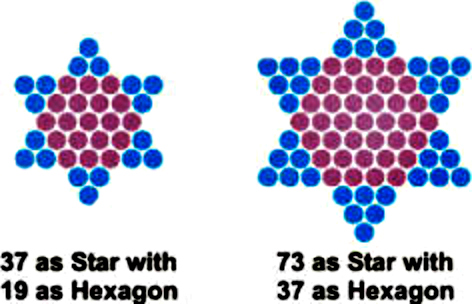Another connection between 19 and 54 and 73, besides 19/73 = .260, the numerical value of the Tetragrammaton (יהוה), is that 19 of the 54 portions of the Torah begin with a word that has either a gematria value, or an ordinal value, of 42. One final redundant connection is that 54 is the sum of the small gematria of the first 5 words of the Torah, while the next two words of the 7-word first verse is 28. The first word is 13.

Before we return to the relationship between the alef-bet and Phi proportions, there are two more equations at the heart of the Torah that establish the indelible connection between Phi and the Torah and illustrate the degree of precision and intent that went into designing the Torah. As first revealed in The Genesis Prayer: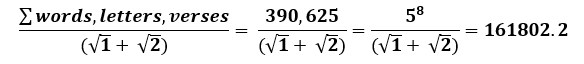If we add the 248 columns in the Torah and the actual number of total rows in the Torah (248 x 42 rows/column less all the blank rows) to the total words, letters and verses, we get a total of 401273 for the 5 separate written divine elements in the Torah, and: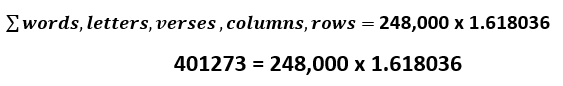How close is that to true Phi? It is about 99.9999% precise, or within 1.0000012 of actual phi.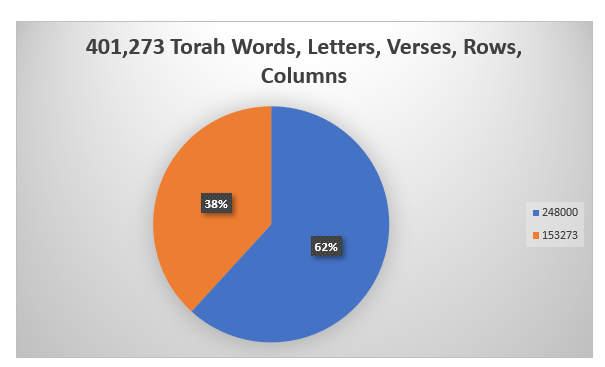Why 248 and 248,000? We do not know, except that 248 is the gematria of Abraham and the sum of the Hebrew Names of each of the 5 Books is 2480, and that there are 248 columns in “The Torah(התורה)” of numerical value 616, whose square root is 24.8….  Maybe it is another way of pointing us in the direction of the 3 portals (1st Shema verse, 1st Torah Verse and 42-Letter Name), whereby 10,000 – their sum (1118 + 2701 + 3701) = 2480 yet again. Moreover, it may be connected to the fact that 2480 = 620 x 4 and that 620 is both the numerical value of Keter(כתר), the highest crowning sefira (dimension), and is representative of the 620 letters in the 10 Commandments, the high point of the Torah. Or maybe it is a deeper way to illustrate to us the connection between the Phi based E 8 Lattice of 248 dimensions and the 58 Torah elements.

Since we have shown that all Torah knowledge tends toward and point to Alef (One) it is not surprising that 248/5 = 111 (Alef), especially considering that the square root of 5 is the key component in Phi. And along those same lines, 8/5 = 3.58, as in the numerical value of Moshiach (358), the Anointed One.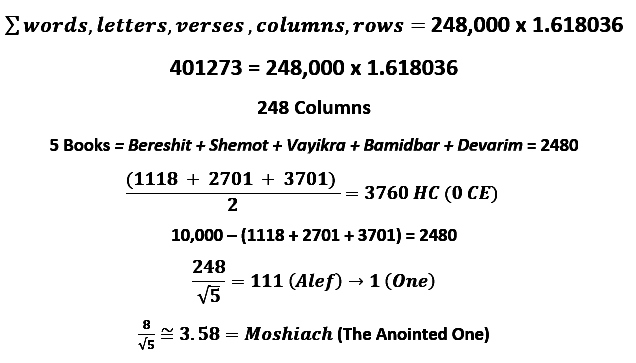Hashem

The word for Name in Hebrew is Shem (שמ) and as we keep circling back to the importance of the connection between the 42=Letter Name, the Torah’s first verse and the Shema, it is noteworthy to mention, as Danny recently showed me, that the sum of the 4 Shins (ש) and 4 Mems (מ) in the Torah’s first verse and in the Shema’s first verse equals 2480, so the 8 Letters  = the 5 Names. Always symmetry, always redundancy, always a reason—it is our job to find it.

Coupled with Name in Hebrew, Shem (שמ), the letter Hey (ה) of numerical value 5, turns Shem into Hashem(השם), referring to G-d. The 5th Name of the 72 Names (Triplets) is (מהש), a permutation of Hashem(השם) and of Moses(משה), as in the 5 Books of Moses. The 5th word in the Torah, H’Shama’im (השמים), meaning Heaven, begins with Hashem(השם), so Heaven can be interpreted as the Sea of Hashem. Five (5) x 345, the numerical value of Hashem and Moses is 1775, the total gematria of the 27 letters of the Hebrew alphabet when the 5 final letters (ךםןףץ) are included. This is also the reason why Moses was accused of stealing the 1775 shekels collected for the building of the Mishkan in pasha Pekudei.

The association between 5 and the 5 Books of Moses and the 54-unit shift and the 54 portions of the Torah is deeper than just being integrated design elements meant to show off the Torah’s Creator’s knowledge of mathematics and the universe.  It has to do with how the Torah is split up, or partitioned, which we’ll get into shortly.  For now, just keep in mind that every Torah element must be divided 5 ways and also 54 ways.

Shavua Tov

The next installment continues shortly as we move out of the introductory phase of this series and into the heart of the matter.

11 thoughts on “Part III Phi and Beyond”

1.Moshe Friedman says:

Wow, this is really a lot of great information to review and digest, however there are a few apparent bloopers that should be fixed.

You wrote:

If we were to apply the Phi proportion to the 1495 total value of the alef-bet, the subordinate values would be split into 924 + 571 = 1495, where a = 974 and b = 571.

— At the end of the sentence there is an obvious typo, and instead of “where a = 974,” it should say “where a = 924.” —

You wrote:

1495 x .6180 = 924

924 x .6180 = 571

1495

— On my computer screen this appears fine (and with the middle line underlined), however on the screen of my android device the second line appears as .924 x .6180 = 5.71 (and also with underlining), with decimal points before the 9 of 924 and between the 5 and 7 of 571, which apparently is some sort of technical glitch… —

You wrote:

Nonetheless, back to that first 28 letter verse of the Torah, the first 5 letters (בראשי) of that verse and thus of the Torah have a collective ordinal value, letter placement within the alphabet value, of (2+20+1+10+21) = 54.

— In the parentheses at the end of the sentence the order of the last two numbers 10 and 21 should be reversed, to correspond to the letters Shin (21) and Yud (10) in the proper order, (2+2+1+21+10) = 54. —

You wrote:

Plotting out those 11 letters, we get:

בראשית־ברא־אל

2,20,1,21,10,22,2,20,1,1,12 and their sum is 112.

First, we combine the letters so you can see what I was seeing:

22012110222201112

Then we break them down to natural syllables:

2201 10 2122 2201 112

BRE Sh YT BRE EL

— The first two lines of these strings of numbers are correct, however in the third line, where you wrote “2201 10 2122 2201 112,” the values for the Sh (Shin) 21, and the Y (Yud) 10, again somehow became reversed, and the proper sequence should instead read “2201 21 1022 2201 112.”

Also it is not clear why the “natural syllables” were split this way, and seemingly the word Breishit should not be split into three syllables, but rather into just two syllables, BRE and ShYT, and the next word Bora should not be expressed as one syllable, but rather it should be split into two syllables, Bo and RE.

These corrections and changes would require a revision of the lessons gained from the conversion from base 3 to base 10. Using the syllable breaks as presented would yield 73 7 35 73 14, which sum to 202, and using syllable breaks as I am suggesting would yield 73 602 2 19 14, which sum to 710.

Also unclear is what you wrote, “If we broke the word sequence into syllables we would get (7+3+6+0+2+7+3) = 28,” since although these digits do add up to 28, however this time your number sequence starts with 73 (7+3) and 602 (6+0+2), as per syllable breaks as I suggested, but then it gets confusing again since you next have BRE (BoRE) as one syllable, 73 (7+3), and you leave out the last syllable EL, 112 (1+1+2). —

You wrote:

Moreover:

2+2+0+1+1+0+2+1+2+2+2+2+0+1+1+1+2 = 22 (letters)

— This is correct, only again the digits corresponding to the Sh (Shin) 2+1 and the Y (Yud) 1+0 are in reverse order, and the proper string of numbers/letters should read 2+2+0+1+2+1+1+0+2+2+2+2+0+1+1+1+2 = 22. —

———-

You wrote:

Though this does not prove the existence of G-d, it does go a long way in proving purposeful design, which can be taken as divine intent.

— This is a very good and important point, but all of the connections to Hashem’s Name and the key aspects of Hashem’s Torah make this evidence even clearer and recognizable as of the “Fingerprint of G-d,” or “Signature of G-d,” as you have written before… —

———-

You wrote:

8/√5 = 3.58, as in the numerical value of Moshiach (358), the Anointed One.

— Actually, more accurately 8/√5 = 3.5777, plus a small fraction more, and thus aside from the rounded off value of 3.58 which hints to Moshiach, the more accurate value may also hint to the year of Moshiach’s predestined arrival, 5778.

The idea of Moshiach is also clearly hinted in the Phi sequence, which starts, 1, 2, 3, 5, 8, and the numbers 3 5 8 hint to the gematria of משיח, Moshiach, 358 (this idea is already noted in Ezra’s book “There’s Nothing Random About the Universe,” at the bottom of page 28 and top of page 29, and probably in many places).

Further, in the numbers in the continuation of the sequence, 1, 2, 3, 5, 8, 13, 21, the 1 hints to Hashem, and then the 2, 3, 5 can be combined as 2 + 3 + 5 = 10, corresponding to the letter י, Yud, the 10th letter of the Alef Beis. The next number 8 corresponds to the letter ח, Ches, the 8th letter of the Alef Beis. The next number 13 corresponds to the letter מ, Mem, the 13th letter of the Alef Beis. The next number 21 corresponds to the letter ש, Shin, the 21st letter of the Alef Beis, and these letters can be rearranged, or read on the graph of the Phi curve, as is proper for Hebrew, from right to left, to spell משיח, Moshiach.

Additionally, starting from the 5th number in the series, the number 8, the sum of the Phi sequence numbers 8 + 13 + 21 + 34 + 55 + 89 + 144 = 364, which this is the same as the gematria of המשיח, HaMoshiach, The Messiah, plus 1 for the Kolel, representing Hashem.

May we continue seeing great revelations, the fulfillment of the Torah’s prophecies, and the culmination of Hashem’s plan, very soon indeed!

2.Jeffrey Meiliken says:

Thanks once again for your valuable help, Moshe. As for the formatting in Android, it was just that the underline between the close numerals gets abbreviated to look like a decimal point. I’ll try to keep that in mind in the future. As for the Yud-Shin reversal, I’d caught that and was still trying to figure out why I made that error as usually, unless I was just tired, there was some divine inspiration that I just wasn’t seeing clearly. You forced my hand (thankfully) and you’ll note on the revised version that indeed there was a reason, which will become much more significant as the series rolls on. While your later points are all very valid the objective in this paper at this stage is not to point to 5778–that is easily made (as you know) later on once newer concepts are proven. As for G-d’s Names, we will get to them in the appropriate time, but first there is much that needs to be laid out and proven first. I know you get it but we need to reach out to the every growing population of people for whom reading G-d’s Names in a “Book” is just religious and means nothing. Their world needs to be rocked and we can only do that by changing their beliefs at their core, not by chipping away at their surface understanding. Your patience is appreciated and your comments and editing invaluable.

3.Peter says:

Wonderful stuff Ezra – Thank you!
The “Uni-Verse”
The 304805 letters can be divided into the 28 letters of Verse 1.

That leaves 304,777 other letters in the Torah. I think of those 304,777 letters as (symbolically or more than that) FOUR sets of 7 (304 (thousand), followed by 7, 7 and 7).

In other words the original code-proram for the creation, 28 letters’ worth, was then expanded into 4 sets of 7 — which we know as the rest of the Torah (now rearranged into the stories we know – Abraham, on t Joseph and his brothers, the Exodus etc . . )

“Unus” the Latin word for One (as in “E Pluribus Unus” ) probably is the source for our English word “Universe” – as in the “One Verse” that was used to create it.

4.Antonio says:

Awaiting your next article with great impatience, Jeff, thank you for all your effort… also wonderful teaching of this teacher… The higuera/Torah already flourished on its sod and the summer KeYTz (Fin) KeTz is close… 13 Mark https://m.youtube.com/user/FacingEachOther/videos

5.Peter says:

Phi In The (Lower) 42 Letter NName

Taking a “zig-zag” pattern through the “Triplets of the 42 Letter Name”, staring Top Right, then Left to the 2nd Row, Right into the 3rd Row . . . .

We have (6 359 57 493 110 187 and 500. They sum to 1618, a representation of “Phi” (1.618 . . .). Leaving the top and bottom Rows out of the sum, we are left with 1206 (1618 less (6+406) = 1206, the value of “The Great Light”, or Aur/Light in which the Aleph is cunted as Eleph (1000). This makes Aleph-Vau-Resh sum to 1000 + 200 + 6 = 1206 rather than the “standad” 207.

Etz (Tree) as in Etz H’Chaim (Tree of Life etc) is spelled – Ayin-Tsadhe, the 16th and 18th Letters of the Aleph-Bet (Aleph-Beis). Perfection, Beauty and Phi in “Tree”.

6.Peter says:

Oops!

We have (6 359 57 493 110 187 and 500 should read “We have (6 359 57 493 110 187 and 406)”. Those numbers DO sum to 1618.

7.Peter says:

Light & Gold

The central (4th out of 7) word of the Torah’s first verse is Aleph-Tau, value 1+400 = 401.

401 is the 79th Prime Number – with 79 being the Atomic Number of Gold.

The 401,273 Torah “elements” are symbolic of that “Golden Prime – Gold in the Temple” link, and 273 = 3 * 7 * 13. Those three Prime Numbers (3, 7, 13) sum to 23, the value of “String ” as in String Theory and the concept of Aur/Light as 207 (1 + 6 + 200) being a 9 Fold String, as in 9 * 23 = 207. Fittingly, 23 is the 9th Prime.

The Central Column of “The Tree” is Keter-Tiphereth-Yesod-Malcuth, values 620, 1081, 80 and 496, summing to 2277 or 11 fold “Light”/207. What is being depicted is Light reaching every corner of the 11 Dimensional Matrix in which our 10 Dimensional Universe sits – hence the 1024/”megabyte” number of word values in the Torah as per Ezra’s writing.
Abrahm (“Mr 248” as in 248 Fundamental Particles, 248 structural components in the (male) human body, CERN and the E-8 Lie Group etc) rejuvenated at age 99 — shades of the 9 Fold String that is Light blasting through the whole Matrix.
When Abraham rejuvenated, Sarai’s name changed to Sarah, value 505. She and her “one”, her one and only husband, became 505 + 1, the 506 value of the top-line entry point into the Lower 42 Letter Name.

8.Jeffrey Meiliken says:

Excellent find. When I circle back to Phi in the series I’m going to include this. It’s a brilliant find.

9.Jeffrey Meiliken says:

We will soon be getting into primes in a much deeper way. Thanks. That E-8 Lie group is definitely not coincidental. The number 23 is key. Thanks again.

10.David Lome says:

Jeff:

Your work is exceptional! Keep it up!

Kodesh Tov,

David

11.Jeffrey Meiliken says:

Thanks. Chodesh Tov